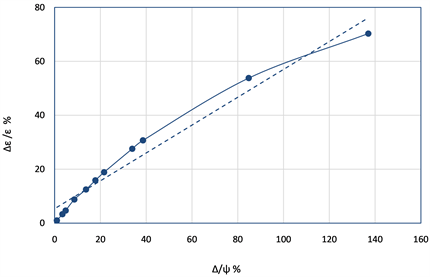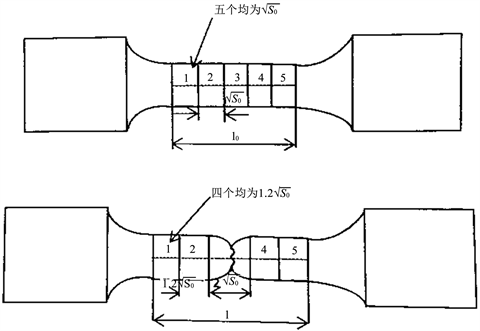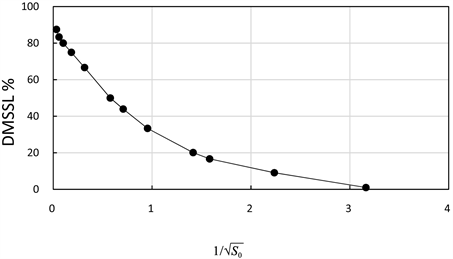#### 期刊菜单

Analysis on Deviation and Necking Formula between the Elongation and Reduction in Tensile Test

Abstract: According to density invariability of elongation and reduction of specimens in the tensile, by adapting formulas we can calculate the mathematical compare relation of them. Conditions to cause necking formula and deviation rules are gained. From deviation relations of reduction and elongation we find that in the case of smaller than 0.09 reduction deviation it will become good with compare to elongation one. In the case of bigger than certain value reduction one of 110 will be good too. They are 1.83 times good to compare with the strain. Additionally the formula of necking is gained with ψ > kε. In general the k will be larger than 2. If the necking becomes big, the k will become big too. It is thought that Twin causes necking and dislocation causes strain.

1. 引言

2. 计算结果

${\epsilon }_{t}=LN\left(1+\epsilon \right)$ (1)

$由于\text{d}{\psi }_{t}=\frac{\text{d}S}{{S}_{0}},所以{\psi }_{t}=\underset{{\text{S}}_{\text{0}}}{\overset{\text{S}}{\int }}\frac{\text{1}}{\text{S}}\text{dS}=LN\frac{S}{{S}_{0}}=LN\frac{{S}_{0}-\Delta S}{{S}_{0}}$ (2)

${\psi }_{t}=LN\left(1-\psi \right)$ (5)

${\psi }_{t}\approx \psi$ (6)

3. 讨论

$\frac{{\text{d}}_{0}}{\text{d}}=\frac{\sqrt{l}}{\sqrt{{l}_{0}}}=\frac{\sqrt{{S}_{0}}}{\sqrt{S}}$ (7)Figure 1. Relation between the true elongation deviation and the reduction deviationTable 1. Deviation of reduction between ψ t = 1.09 ψ and ψ t = 2.09 ψFigure 2. Variation of the specimen elongation before and after test

$\psi =\frac{\Delta {l}_{j}}{{l}_{0}}\ge \epsilon /2$ (8)

ψ和 $1/\sqrt{{S}_{0}}$ 的关系曲线如图3。断面收缩率随着 $1/\sqrt{{S}_{0}}$ 减少，ψ从90%减少到0。 $1/\sqrt{{S}_{0}}$ 变小则试件面积S0变大断面收缩率变小。收缩率变大第一是因为试件尺寸外的外部条件，这时材料承载大，颈缩也大。第二是因为内部结构问题，这时裂纹经过的路径多而产生颈缩。这是试验负载和材料性质所致。从缺陷分析转位引起延伸率增加，双晶引起收缩率增加。Figure 3. Relation of the reduction and $1/\sqrt{{S}_{0}}$

4. 结论

1) 在真实收缩率小于1.09倍ψ时真实延伸率和延伸率偏差之比小于断面收缩率偏差率之比，收缩率更真实。当真实收缩率大于2.09ψ时收缩率偏差又变大，当真实收缩率一定时收缩率变大，偏差也变大，收缩率也更真实。真实收缩率在0.09和1.09偏差时后者是前者的近2倍，说明收缩率值在偏差增大后变得更真实。当 $\Delta /\psi$ 为80时收缩率偏差比延伸率的偏差大，约1.83倍，说明收缩率比延伸率更真实1.83倍。

2) 对于延性材料延伸试件引起颈缩的范围为断面收缩率 $\psi \ge 2\epsilon$$\Delta {l}_{j}/{l}_{0}\ge \epsilon /2$ ，颈缩越大 $\Delta {l}_{j}/{l}_{0}$ 越大。当 $\Delta {l}_{j}/{l}_{0}\ge \epsilon$ 时整个收缩率 $\psi \ge 3/2\epsilon$ 或达到 $\psi \ge 2\epsilon$。随着颈缩继续变大 $\psi \ge K\epsilon$ ，此时 $K\ge 2$ ，甚至 $K\ge 7$。我们认为转位引起延伸率的增大，双晶引起收缩率增加。因为拉断时材料在外力或动载作用下会出现大的响声，由此判断一定数量甚至大量的双晶出现，我们认为它是颈缩失稳的主要原因。

KOSEF (the Korea of Science and Engineering Fund) under the Specified base program 96-0300-11-01-3.

  刘鸿文. 材料力学[M]. 第二版. 北京: 高等教育出版社, 1982: 29.  许润, 许甫宁, 林水根, 金然勗. 拉伸试验中矩形和圆截面试件的延伸率和断面收缩率之间的数学关系[J]. 中文科技期刊数据库自然科学(全文版), 2018(7): 101.  宋玉泉, 索忠林, 管志平, 刘颖. 材料参数对拉伸失稳影响的力学解析[J]. 金属学报, 2006, 42(4): 338.